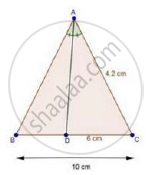Share

# In a δAbc, Ad is the Bisector of ∠A, Meeting Side Bc at D. If Ac = 4.2 Cm, Dc = 6 Cm and 10 Cm, Find Ab - Mathematics

Course

#### Question

In a ΔABC, AD is the bisector of ∠A, meeting side BC at D.

If AC = 4.2 cm, DC = 6 cm and 10 cm, find AB

#### SolutionWe have,

BC = 10 cm, DC = 6 cm and AC = 4.2 cm

∴ BD = BC – DC = 10 – 6 = 4 cm

⇒ BD = 4 cm

In ΔABC, AD is the bisector of ∠A.

We know that, the internal bisector of an angle of a triangle divides the opposite side internally in the ratio of the sides containing the angle.

therefore"BD"/"DC"="AB"/"AC"

rArr4/6="AB"/4.2        [∵ BD = 4 cm]

⇒ AB = 2.8 cm

Is there an error in this question or solution?

#### APPEARS IN

RD Sharma Solution for Class 10 Maths (2018 (Latest))
Chapter 7: Triangles
Ex. 7.3 | Q: 1.5 | Page no. 31
RD Sharma Solution for Class 10 Maths (2018 (Latest))
Chapter 7: Triangles
Ex. 7.3 | Q: 1.5 | Page no. 31